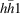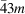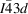International
Tables for
Crystallography
Volume B
Reciprocal space
Edited by U. Shmueli

International Tables for Crystallography (2010). Vol. B, ch. 2.5, pp. 334-344   | 1 | 2 |

## Section 2.5.3.3.6. Examples of space-group determination

M. Tanakaf

#### 2.5.3.3.6. Examples of space-group determination

| top | pdf |

A simple example of point-group determination has already been given for Si in Section 2.5.3.2.5. In this section, two examples of space-group determination for rutile and samarium selenide are described, in which the point-group determination still accounts for an important part. The examples look to be a little sophisticated but are a good exercise for those who want to acquire experience in CBED space-group determination. The present determination is carried out by assuming the lattice parameters to be known.

Rutile (TiO2). The space group of rutile is well known to be P42/mnm. The lattice parameters are a = b = 0.459 nm and c = 0.296 nm. Fig. 2.5.3.15(a) shows a CBED pattern taken with the  incidence at an accelerating voltage of 80 kV. Since no fine HOLZ lines appear in all the discs, projection diffraction groups (column VI of Table 2.5.3.3) have to be applied to explain this pattern. The projection (proj.) WP shows symmetry 4mm. The projection diffraction group is found to be 4mm1R from Table 2.5.3.3. Thus, possible diffraction groups are 4mRmR, 4mm, 4RmmR and 4mm1R. Another CBED pattern at a second crystal orientation needs to be taken because Fig. 2.5.3.15(a) shows only projection symmetry. Figs. 2.5.3.15(b) and (c) show CBED patterns taken with the  incidence at an accelerating voltage of 100 kV. In Fig. 2.5.3.15(b), which is the central part of Fig. 2.5.3.15(c), no HOLZ lines are seen. The symmetries of the projection BP and projection WP are both 2mm. The projection diffraction group of the pattern is 2mm1R. The WP of Fig. 2.5.3.15(c) is seen to have one mirror symmetry m. The diffraction groups which satisfy symmetry m are m, m1R and 2RmmR. Among these diffraction groups, the diffraction group whose projection becomes 2mm1R is only diffraction group 2RmmR. By consulting Fig. 2.5.3.4, diffraction group 2RmmR obtained from Figs. 2.5.3.15(b) and (c) and one diffraction group 4mm1R among diffraction groups 4mRmR, 4mm, 4RmmR and 4mm1R obtained from Fig. 2.5.3.15(a) commonly satisfy point group 4/mmm. Thus, the point group of rutile is determined to be 4/mmm.Figure 2.5.3.15 | top | pdf |CBED patterns of rutile. The procedures for identifying the symmetry are also shown. (a)  incidence at 80 kV: the projection (proj.) WP shows symmetry 4mm. (b, c)  incidence at 100 kV: the projection BP and projection WP show symmetry 2mm and the WP shows symmetry m (the point group is 4/mmm). (d) Spot diffraction pattern showing no extinction caused by the lattice type (lattice type P). (e) Near- incidence at 100 kV to excite exactly the 001 reflection: no extinction lines in the 001 disc (space group P42/mnm).

Fig. 2.5.3.15(d) shows an ordinary diffraction pattern taken with the  incidence at an accelerating voltage of 80 kV. With the help of the lattice parameters and the camera length, the indices of the reflections are given as shown in the figure. There are no kinematically forbidden reflections. Thus, the lattice type is determined to be primitive P.

Possible space groups which satisfy point group 4/mmm and primitive lattice type P are those of Nos. 123–138 in Table 2.5.3.9. In Fig. 2.5.3.15(a), the dynamical extinction line A2 is seen in the 100 disc and in the equivalent 010 disc. By consulting Table 2.5.3.9, four space groups P4/mbm, P4/mnc, P42/mbc and P42/mnm are selected. In Fig. 2.5.3.15(b), the dynamical extinction line A2 is seen in the 010 disc but not in thedisc. Two space groups P4/mnc and P42/mnm are selected from the four. To distinguish the two space groups, it is found from Table 2.5.3.9that a CBED pattern taken with the  electron incidence should be examined. Fig. 2.5.3.15(e) shows a CBED pattern taken with the  incidence at 100 kV, where the 001 reflection is exactly excited. Thereflections are kinematically allowed for space group P42/mnm but kinematically forbidden for P4/mnc. Since in the case of P4/mnc, no Umweganregung (multiple scattering) paths to the 001 reflection exist in the zeroth-order Laue zone, only the intensities of HOLZ lines, which are caused by Umweganregung via HOLZ reflections, are expected to appear in the 001 disc. If such Umweganregung is not practically excited, the 001 reflection must have no intensity. However, strong intensity produced by two-dimensional interaction is seen in the 001 disc of Fig. 2.5.3.15(e). This indicates that the reflection is an allowed reflection. Therefore, the space group of rutile is determined to be P42/mnm, which agrees with the space group already known.

Samarium selenide (Sm3Se4). Sm3Se4 has the Th3P4 structure type with space groupat high temperatures. The lattice parameters are a = b = c = 0.8885 nm. It was expected that Sm3Se4 would transform to an ordered state of electrons with two valences of +2 and +3 around 150 K. The determination of the space group of the material was conducted at 100 K and room temperature. The space groups at both temperatures were determined by CBED to be the same. The following experiments were performed at 100 K.

Fig. 2.5.3.16(a) shows a CBED pattern taken with the  incidence at 80 kV, which clearly shows the first-order-Laue-zone reflections. The symmetry of the WP is seen to be 3m with the help of the enlarged insets. Possible diffraction groups are 3m, 3m1R and 6RmmR from Table 2.5.3.3. Fig. 2.5.3.16(b), which is the central part of Fig. 2.5.3.16(a), shows projection symmetry 3m, indicating that the projection diffraction group is 3m1R. Among the three groups 3m, 3m1R and 6RmmR, diffraction groups for which the projection diffraction group is 3m1R are 3m and 3m1R. Possible point groups are found to be 3m,andfrom Fig. 2.5.3.4. Fig. 2.5.3.16(c) shows a CBED pattern taken with the  incidence at 80 kV. The WP is seen to have symmetry 2mm. Allowed diffraction groups are 2mm, 2mm1R and 4RmmR. Fig. 2.5.3.16(d), which is the central part of Fig. 2.5.3.16(c), shows projection WP symmetry 4mm, indicating that the projection diffraction group is 4mm1R. The diffraction group among the three groups 2mm, 2mm1R and 4RmmR whose projection diffraction group is 4mm1R is 4RmmR. Possible point groups are found to beandfrom Fig. 2.5.3.4. Thus, the point group which satisfies the results obtained at the two crystal orientations is.Figure 2.5.3.16 | top | pdf |CBED patterns of Sm3Se4. The procedures for identifying the symmetry are also shown. (a, b)  incidence at 80 kV: the WP symmetry is 3m (a) and the projection (proj.) WP symmetry is 3m (b). (c, d)  incidence at 80 kV: the WP symmetry is 2mm (c) and the projection WP symmetry is 4mm (d). Dynamical extinction lines A2 and A3 are seen (d). The point group is determined to be. (e) Spot diffraction pattern taken with the  incidence at 80 kV shows the absence of 0kl reflections. The lattice type is determined to be I. (f)  incidence at 100 kV: dynamical extinction lines A in HOLZ reflections confirm the existence of a glide plane. The space group is determined to be.

Fig. 2.5.3.16(e) shows an ordinary diffraction pattern taken with the  incidence at 80 kV. With the help of the lattice parameters and the camera length, the indices of the reflections are given as shown in the figure. The reflections 0kl (k + l = 2n + 1) are found to be kinematically forbidden. Thus, the lattice type is determined to be I.

The space groups having point groupand lattice type I areandfrom Table 2.5.3.9. Fig. 2.5.3.16(d) shows dynamical extinction lines A2 in the 033 disc and equivalent discs (also broad lines A2 in the 011 discs). Since the former space group does not give any dynamical extinction lines, the space group is determined to be. For confirmation, a CBED pattern which contains the second-order-Laue-zone reflections was taken (Fig. 2.5.3.16f). Dynamical extinction lines A are seen in the 2,22,22 disc and the equivalent discs. This result also identifies the space group to be notbutwith the aid of Table 2.5.3.12.